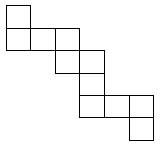The ribbon rule

Ribbon operators and homogeneous (Hall-Littlewood) symmetric functionsThis picture above represents a ribbon (drawn in cartesian rather than matrix notation). Starting from left to right (and top to bottom) number the cells 1 to n where n is the total number of cells. The numbers i where the i+1st cell is below the i-th cell are the descents of a ribbon. The descent set of a ribbon R is the set of i such that i is a descent of R. The descent set of the ribbon above is {1,4,6,7,10} and it is a ribbon of size 11.

Observation 1: The descent set of a ribbon is a subset of {1..n-1} where n is the length of the ribb
on.

Observation 2: The ribbon is determined by its descent set and size (i.e. the ribbons of size n are in bijection with the subsets of {1..n-1}).

Observation 3: A ribbon is also a skew partition. If the outer partition is \la = (\la_1, \la_2, ..., \la_k) then the inner partition is \la^{rc} = (\la_2-1, \la_3-1, ..., \la_k -1). In the example above \la = (6,6,4,4,3,1) and \la^{rc} = (5,3,3,2).

Uses the package SF

> with(SF):

Warning, new definition for conjugate

An operator which adds a column to the left of a partition indexing the Schur function (Sw) followed by an operator that adds a sequence of columns (in the shape of a partition \nu).

> eee:=k->if k=0 then 1; elif k<0 then 0 else top(e.k) fi:

> Sw:=proc(m,EX) local degz, PEX, PEXz, rslt,i;
degz:=SF/stdeg(EX);
PEX:=top(EX);
PEXz:=expand(subs({seq(p.k=p.k+z^k,k=1..degz)},PEX));
rslt:=PEX*eee(m);
for i from 1 to degz do
rslt:=rslt+(-1)^i*coeff(PEXz,z,i)*eee(m+i);
od;
tos(rslt);
end:

> Swnu:=proc(nu,EX) local out,nup,i;
nup:=SF/conjugate(nu);
out:=EX;
for i from nops(nup) to 1 by -1 do
out:=Sw(nup[i],out);
od;
out;
end:

Test Sw and Swnu:

The function ribop takes a subset and an n, creates the operator that adds columns in the outer shape of the ribbon and then applys a skew operator in the shape of the inner partition of the ribbon.

> ribop:=proc(EX,sbset,n) local i,cnt,rslt,partage;
cnt:=1;
rslt:=EX;
partage:=NULL;
for i from n-1 to 1 by -1 do
if not member(i,sbset) then
rslt:=Sw(cnt,rslt);
if cnt>1 then partage:=cnt-1,partage; fi;
else
cnt:=cnt+1;
fi;
od;
tos(skew(s[op(SF/conjugate([partage]))],Sw(cnt,rslt)));
end:

> tau:=sbset->convert(sbset,+):

h1m adds a column of size m on a homogeneous symmetric function if the partition has length less than or equal to m. It is the sum over all ribbon operators of size m.

> h1m:=proc(m,EX) local ss,out;
if m=1 then Sw(1,EX);
else
out:=0;
for ss in combinat[powerset](m-1) do
out:=out+ribop(EX,ss,m);
od;
tos(out);
fi;
end:

H1mt adds a column of size m on the Hall-Littlewood symmetric functions if the indexing partition has length less than or equal to m. It is the sum over all ribbon operators of size m times t^{\sum i} where the sum is over all i that are not a descent of the ribbon.

> H1mt:=proc(m,EX) local ss,mc2,out;
if m=1 then Sw(1,EX);
else
mc2:=m*(m-1)/2;
out:=0;
for ss in combinat[powerset](m-1) do
out:=out+t^(mc2-tau(ss))*ribop(EX,ss,m);
od;
tos(out);
fi;
end:

Test ribop, h1m and H1mt

lambda is a function to do a substitution of the form p.k -> f(k) for each k

> lambda:=proc (expr, f) local poly, d, j, res;
poly := top(expr);
d := varset(poly,'p');
res := collect(subs({seq(cat('p',j) = f(j),j = 1 .. d)},
poly),[seq(cat('p',j),j = 1 .. d)],distributed);
map(factor,%);
subs(s[] = 1,%);
end:

ribopq is a q deformation of ribop. ribopq = (ribop^Y P[q X + (1-q)Y]) |_{Y=X}

> ribopq:=proc(EX,sbset,n) option remember;
ribop(lambda(EX,k->q^k*pp.k+(1-q^k)*p.k),sbset,n);
tos(subs({seq(pp.k=p.k,k=1..SF/stdeg(EX))},%));
end:

H1mqt adds a column on a Macdonald symmetric function that is indexed by a
partition of length less than or equal to m.

> H1mqt:=proc(m,EX) local ss,mc2,out;
if m=1 then Sw(1,EX);
else
mc2:=m*(m-1)/2;
out:=0;
for ss in combinat[powerset](m-1) do
out:=out+t^(mc2-tau(ss))*ribopq(EX,ss,m);
od;
tos(out);
fi;
end:

Test ribopq and H1mqt

>